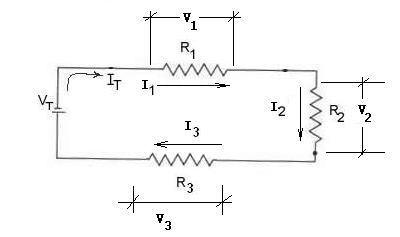#### Series Circuits Analysis

From our studies of series circuits in the laboratory we found that they have several important characteristics.Circuit Legend:
• VT is the Total Voltage
• IT is the total Current
• V1V2, and V3 are the voltage drops across R1R2, and  R3 respectively.
• I1I2, and I3 are the currents through R1R2, and  R3 respectively.
Series Circuits Facts:
• Voltage: The sum of all the voltage drops adds up to the total (source) voltage  VT = V1 + V2 +V3
• Current: The current is always the same IT  = I1 = I2 = I3
• Resistance: The Total resistance RT  can be calculated in two possible ways:
1. RT = R1 + R2 +  R3
2. RT  = VT / IT    (Ohm's Law)
• Power: The Total Power PT  can be calculated in two possible ways:
1. PT = P1 + P2 + P3
2. PT  = VT  IT

Example:
In the circuit above, find all the unknown quantities:
 GIVEN FIND FIND VTotal  = VT = 100 V IT= ? RT= ? V2 = 50 V V1 = R 3= ? R2 = 5.0 Ω V3 = P1 = ? R1 = 1.0 Ω I1 = ? P2 = ? I2= 10 A I3 = ? PT = ?
Solution:
(i)  If  I2= 10 A, Then  I1 =  I= I= I10 A  (Current is constant in a series circuit)
(ii) FOR R1        V1 = I1  x R= 10A x 1.0 Ω  = 10 V
(iii) FOR V3    We know that V= V1 + V2 +V3

Therefore   100 V = 10V + 50V + V3

V= 100V - 5-V - 10 V =  40V
(iv) Now we can find R 3
R 3  = V 3 / I  =  40V / 10A = 4 Ω
(v) Find  the Total Resistance RT
1. Method #1 -----  RT = R1 + R2 +  R3   = 1.0 Ω  + 5 Ω  + 4 Ω  = 10Ω

2. Method #2 ------ RT  = VT / IT      (Ohm's Law)
=  100 V / 10 A  = 10 Ω
You can see that both methods yield the same result
(vi)   Find  P1       P1 =  V1 x  I= 10 V x 10 A = 100 W
This is the power used by R1
(vii)  Find  P2       P2 =  V2 x  I= 50 V x 10 A = 500 W
This is the power used by R2
(viii)  Find  P3      P3 =  V3 x  I= 40 V x 10 A = 400 W
This is the power used by R3
(ix)   Find  PT       PT =  VT x  I= 100 V x 10 A = 1 000 W

This is the total power used by all three resistors together

Or, Alternatively, PT = P1 + P2 + P3  = 100 W + 500 W + 400 W = 1 000 W
You can see that both methods yield the same results